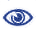# The Prague Stringology Conference 2009

An Efficient Algorithm for Approximate Pattern Matching with Swaps

 Abstract: The Pattern Matching problem with Swaps consists in finding all occurrences of a pattern P in a text T, when disjoint local swaps in the pattern are allowed. In the Approximate Pattern Matching problem with Swaps one seeks to compute, for every text location with a swapped match of P, the number of swaps necessary to obtain a match at the location. In this paper, we present new efficient algorithms for the Approximate Swap Matching problem. In particular, we first present a O(nm2) algorithm, where m is the length of the pattern and n is the length of the text, which is a variation of the Backward-Cross-Sampling algorithm, a recent solution to the swap matching problem. Subsequently, we propose an efficient implementation of our algorithm, based on the bit-parallelism technique. The latter solution achieves a O(mn)-time and O(σ)-space complexity, where σ is the dimension of the alphabet. From an extensive comparison with some of the most recent and effective algorithms for the approximate swap matching problem, it turns out that our algorithms are very flexible and achieve very good results in practice.

 Download paper:PostScript PDF BibTeX reference
 Download presentation: